## More Vector Spaces; Isomorphism

The idea of a vector space can be extended to include objects that you would not initially consider to be ordinary vectors. Matrix spaces. Consider the set M 2x3( R) of 2 by 3 matrices with real entries. This set is closed under addition, since the sum of a pair of 2 by 3 matrices is again a 2 by 3 matrix, and when such a matrix is multiplied by a real scalar, the resulting matrix is in the set also. Since M 2x3( R), with the usual algebraic operations, is closed under addition and scalar multiplication, it is a real Euclidean vector space. The objects in the space—the “vectors”—are now matrices.

Since M 2x3( R) is a vector space, what is its dimension? First, note that any 2 by 3 matrix is a unique linear combination of the following six matrices: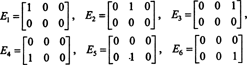Therefore, they span M 2x3( R). Furthermore, these “vectors” are linearly independent: none of these matrices is a linear combination of the others. (Alternatively, the only way k 1 E 1 + k 2 E 2 + k 3 E 3 + k 4 E 4 + k 5 E 5 + k 6 E 6 will give the 2 by 3 zero matrix is if each scalar coefficient, k i , in this combination is zero.) These six “vectors” therefore form a basis for M 2x3( R), so dim M 2x3( R) = 6.

If the entries in a given 2 by 3 matrix are written out in a single row (or column), the result is a vector in R 6. For example,The rule here is simple: Given a 2 by 3 matrix, form a 6‐vector by writing the entries in the first row of the matrix followed by the entries in the second row. Then, to every matrix in M 2x3( R) there corresponds a unique vector in R 6, and vice versa. This one‐to‐one correspondence between M 2x3( R) and R 6is compatible with the vector space operations of addition and scalar multiplication. This means that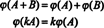The conclusion is that the spaces M 2x3( R) and R 6 are structurally identical, that is, isomorphic, a fact which is denoted M 2x3( R) ≅ R 6. One consequence of this structural identity is that under the mapping ϕ—the isomorphism—each basis “vector” E i given above for M 2x3( R) corresponds to the standard basis vector e i for R 6. The only real difference between the spaces R 6 and M 2x3( R) is in the notation: The six entries denoting an element in R 6 are written as a single row (or column), while the six entries denoting an element in M 2x3( R) are written in two rows of three entries each.

This example can be generalized further. If m and n are any positive integers, then the set of real m by n matrices, M mxn ( R), is isomorphic to R mn , which implies that dim M mxn ( R) = mn.

Example 1: Consider the subset S 3x3( R) ⊂ M 3x3( R) consisting of the symmetric matrices, that is, those which equal their transpose. Show that S 3x3( R) is actually a subspace of M 3x3( R) and then determine the dimension and a basis for this subspace. What is the dimension of the subspace S nxn ( R) of symmetric n by n matrices?

Since M 3x3( R) is a Euclidean vector space (isomorphic to R 9), all that is required to establish that S 3x3( R) is a subspace is to show that it is closed under addition and scalar multiplication. If A = A T and B = B T, then ( A + B) T = A T + B T = A + B, so A + B is symmetric; thus, S 3x3( R) is closed under addition. Furthermore, if A is symmetric, then ( kA) T = kA T = kA, so kA is symmetric, showing that S 3x3( R) is also closed under scalar multiplication.

As for the dimension of this subspace, note that the 3 entries on the diagonal (1, 2, and 3 in the diagram below), and the 2 + 1 entries above the diagonal (4, 5, and 6) can be chosen arbitrarily, but the other 1 + 2 entries below the diagonal are then completely determined by the symmetry of the matrix:Therefore, there are only 3 + 2 + 1 = 6 degrees of freedom in the selection of the nine entries in a 3 by 3 symmetric matrix. The conclusion, then, is that dim S 3x3( R) = 6. A basis for S 3x3( R) consists of the six 3 by 3 matrices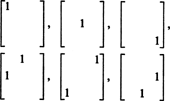In general, there are n + ( n − 1) + … + 2 + 1 = ½ n( n + 1) degrees of freedom in the selection of entries in an n by n symmetric matrix, so dim S nxn ( R) = 1/2 n( n + 1).

Polynomial spaces. A polynomial of degree n is an expression of the formwhere the coefficients a i are real numbers. The set of all such polynomials of degree ≤ n is denoted P n . With the usual algebraic operations, P n is a vector space, because it is closed under addition (the sum of any two polynomials of degree ≤ n is again a polynomial of degree ≤ n) and scalar multiplication (a scalar times a polynomial of degree ≤ n is still a polynomial of degree ≤ n). The “vectors” are now polynomials.

There is a simple isomorphism between P n and R n+1 :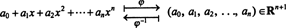This mapping is clearly a one‐to‐one correspondence and compatible with the vector space operations. Therefore, P n R n+1 , which immediately implies dim P n = n + 1. The standard basis for P n , { 1, x, x 2,…, x n }, comes from the standard basis for R n+1 , { e 1, e 2, e 3,…, e n+1 }, under the mapping ϕ −1: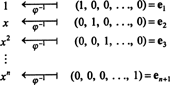Example 2: Are the polynomials P 1 = 2 − x, P 2 = 1 + x + x 2, and P 3 = 3 x − 2 x 2 from P 2 linearly independent?

One way to answer this question is to recast it in terms of R 3, since P 2 is isomorphic to R 3. Under the isomorphism given above, p 1 corresponds to the vector v 1 = (2, −1, 0), p 2 corresponds to v 2 = (1, 1, 1), and p 3 corresponds to v 3 = (0, 3, −2). Therefore, asking whether the polynomials p 1, p 2, and p 3 are independent in the space P 2 is exactly the same as asking whether the vectors v 1, v 2, and v 3 are independent in the space R 3. Put yet another way, does the matrix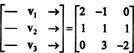have full rank (that is, rank 3)? A few elementary row operations reduce this matrix to an echelon form with three nonzero rows:Thus, the vectors—either v 1, v 2, v 3, are indeed independent.

Function spaces. Let A be a subset of the real line and consider the collection of all real‐valued functions f defined on A. This collection of functions is denoted R A . It is certainly closed under addition (the sum of two such functions is again such a function) and scalar multiplication (a real scalar multiple of a function in this set is also a function in this set), so R A is a vector space; the “vectors” are now functions. Unlike each of the matrix and polynomial spaces described above, this vector space has no finite basis (for example, R A contains P n for every n); R A is infinite‐dimensional. The real‐valued functions which are continuous on A, or those which are bounded on A, are subspaces of R A which are also infinite‐dimensional.

Example 3: Are the functions f 1 = sin 2 x, f 2 = cos 2 x, and f 3 f 3 ≡ 3 linearly independent in the space of continuous functions defined everywhere on the real line?

Does there exist a nontrivial linear combination of f 1, f 2, and f 3 that gives the zero function? Yes: 3 f 1 + 3 f 2f 30. This establishes that these three functions are not independent.

Example 4: Let C 2( R) denote the vector space of all realvalued functions defined everywhere on the real line that possess a continuous second derivative. Show that the set of solutions of the differential equation y” + y = 0 is a 2‐dimensional subspace of C 2( R).

From the theory of homogeneous differential equations with constant coefficients, it is known that the equation y” + y = 0 is satisfied by y 1 = cos x and y 2 = sin x and, more generally, by any linear combination, y = c 1 cos x + c 2 sin x, of these functions. Since y 1 = cos x and y 2 = sin x are linearly independent (neither is a constant multiple of the other) and they span the space S of solutions, a basis for S is {cos x, sin x}, which contains two elements. Thus,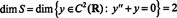as desired.